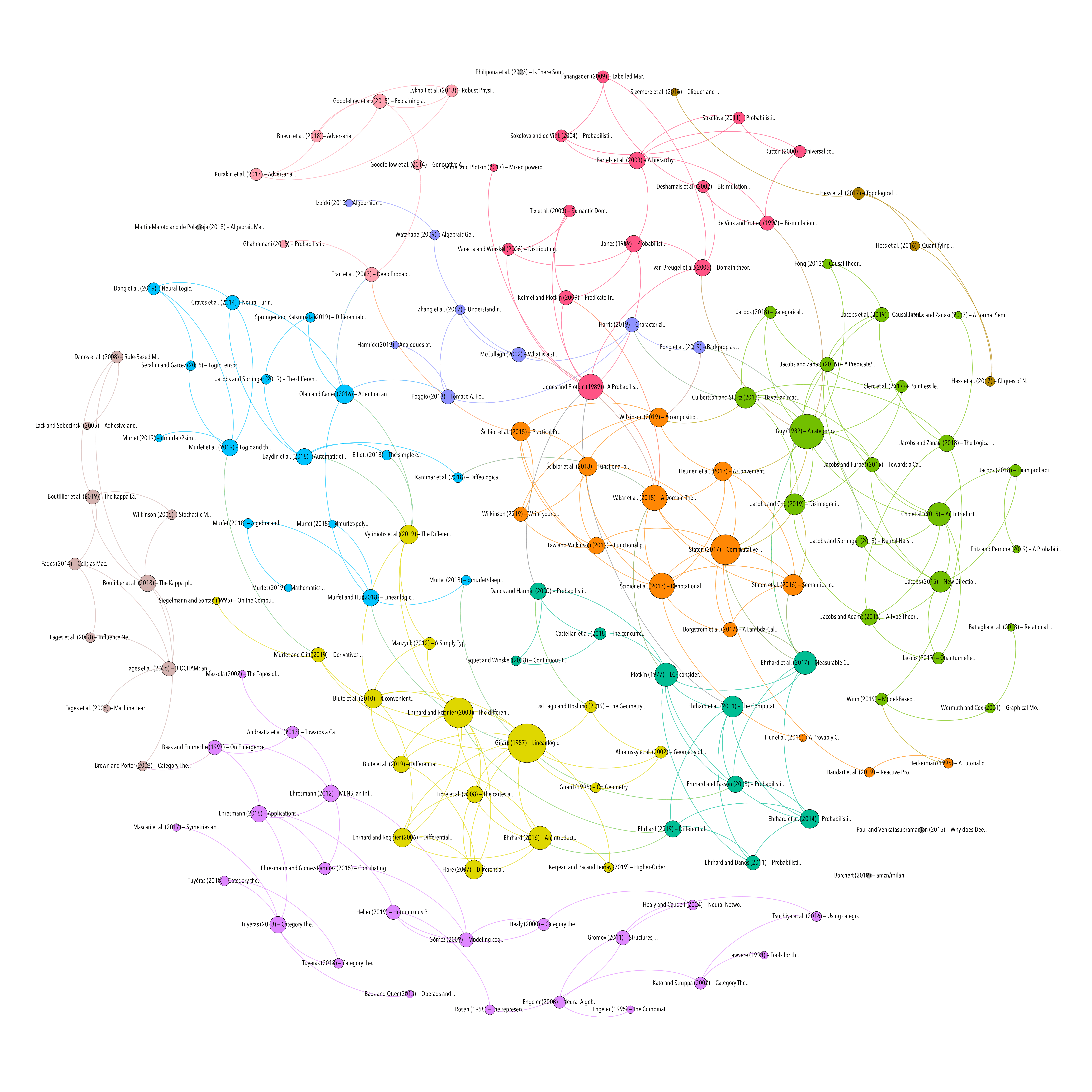# Full bibliography

## The differential calculus of causal functions

Resource type
Authors/contributors
Title
The differential calculus of causal functions
Abstract
Causal functions of sequences occur throughout computer science, from theory to hardware to machine learning. Mealy machines, synchronous digital circuits, signal flow graphs, and recurrent neural networks all have behaviour that can be described by causal functions. In this work, we examine a differential calculus of causal functions which includes many of the familiar properties of standard multivariable differential calculus. These causal functions operate on infinite sequences, but this work gives a different notion of an infinite-dimensional derivative than either the Fr\'echet or Gateaux derivative used in functional analysis. In addition to showing many standard properties of differentiation, we show causal differentiation obeys a unique recurrence rule. We use this recurrence rule to compute the derivative of a simple recurrent neural network called an Elman network by hand and describe how the computed derivative can be used to train the network.
Publication
arXiv:1904.10611 [cs]
Date
2019-04-23
Accessed
2019-11-21T20:41:46Z
Library Catalog
Extra
ZSCC: 0000001 arXiv: 1904.10611
Citation
Jacobs, B., & Sprunger, D. (2019). The differential calculus of causal functions. ArXiv:1904.10611 [Cs]. Retrieved from http://arxiv.org/abs/1904.10611
DIFFERENTIAL CALCULUS
Topic
Attachment
Processing time: 0.01 secondsGraph of references (from Zotero to Gephi via Zotnet with this script)